Question

# Given h(t)=(e-t+e-3t)u(t) find: A) The transfer function H(s). B) The locations of all poles and zeros....

Given h(t)=(e-t+e-3t)u(t) find:

A) The transfer function H(s).

B) The locations of all poles and zeros.

C) Determine if the system is stable or not

D) Find the differential equation for this system.

Given problem has been solved with proper explanation and calculations. To understand this problem, a basic knowledge of impulse response of a system, transfer function of a system, differential equation representation of a system and Laplace transformation is required. If you have any doubt, please comment.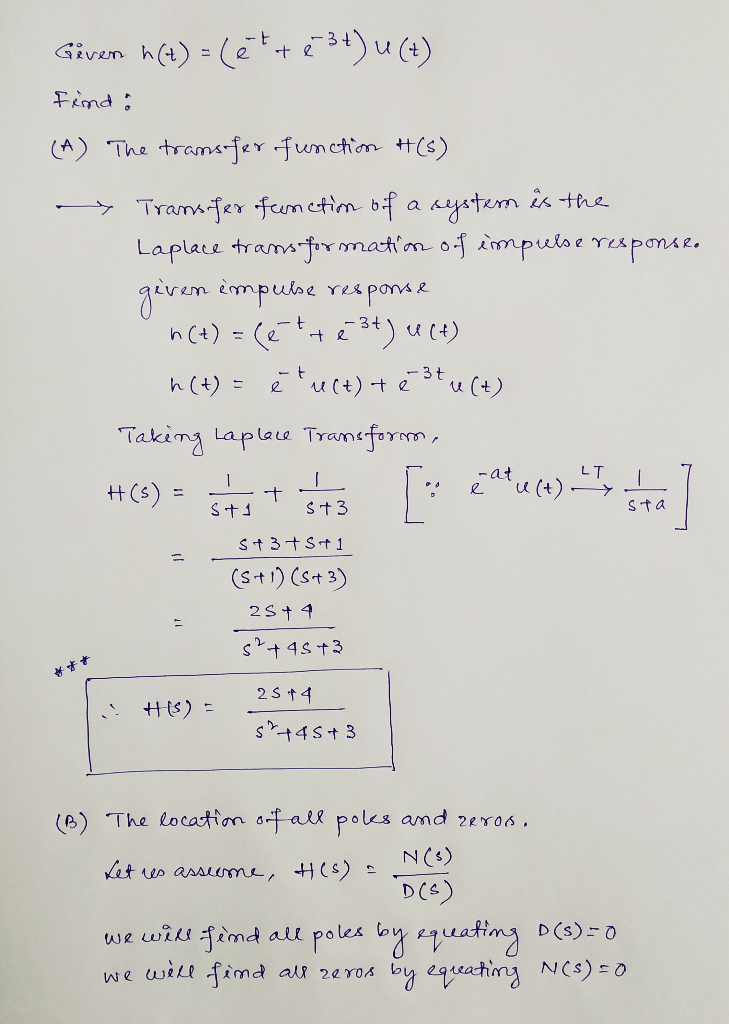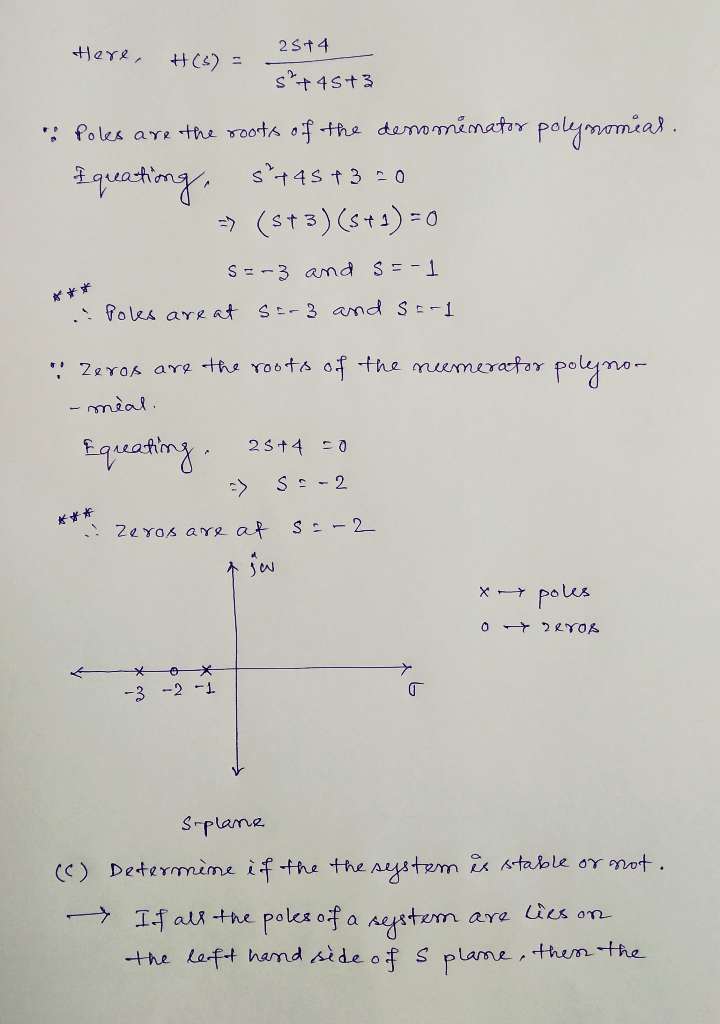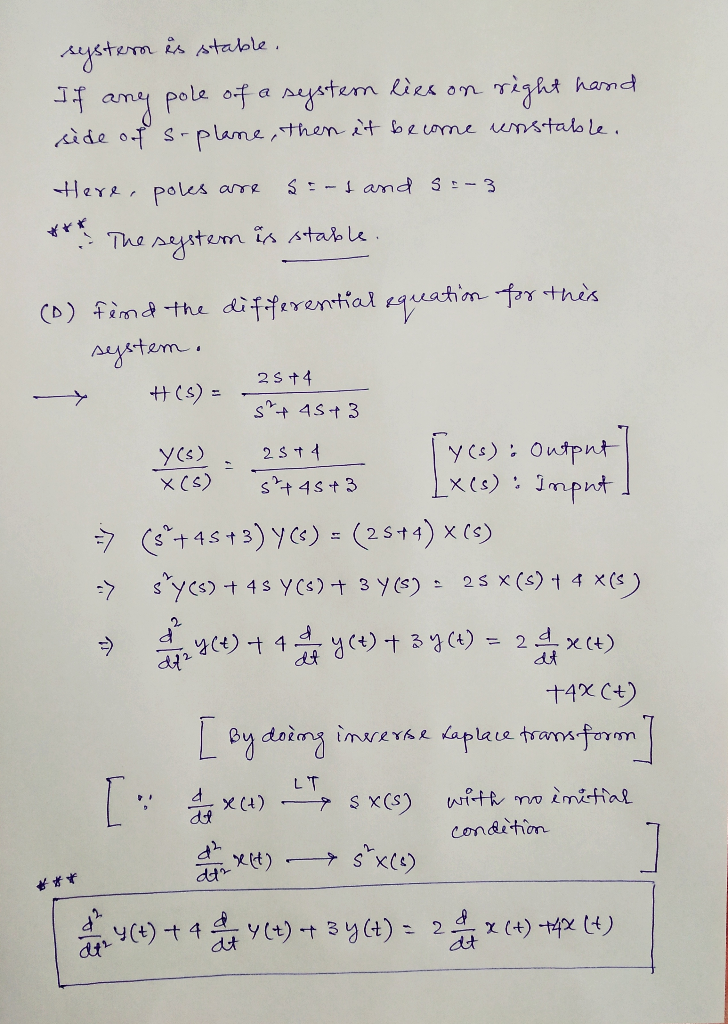#### Earn Coins

Coins can be redeemed for fabulous gifts.

Similar Homework Help Questions
• ### Poles and Zeros For the transfer function given: 0.85 8-44.64 G(s) = 긁+0.83 12.00 Part A-Poles Find the system pole 8 Submit Part B-Poles Find the system pole s2 Submit Part C-Zeros Find the system...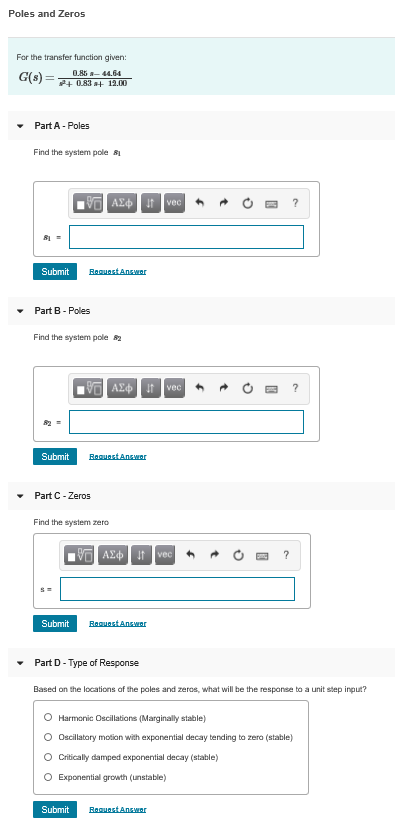Poles and Zeros For the transfer function given: 0.85 8-44.64 G(s) = 긁+0.83 12.00 Part A-Poles Find the system pole 8 Submit Part B-Poles Find the system pole s2 Submit Part C-Zeros Find the system zero Submit Part D-Type of Response Based on the locations af the poles and zeros, what will be the response to a unit step inpue? O Harmonic Oscillations (Marginally stable) Oscillatory motion with exponential decay tending to zero (stable O Critically damped exponential decay (stable)...

• ### a continuous time causal LTI system has a transfer function: H(s)=(s+3)/(s^2 +3s +2) a) find the...

a continuous time causal LTI system has a transfer function: H(s)=(s+3)/(s^2 +3s +2) a) find the poles and zeros b) indicate the poles and the zeros on the s-plane indicate the region of convergence (ROC) c) write the differential equation of the system. d) determine the gain of the system at dc (ie the transfer function at w=0) e) is the system described by H(s) stable? explain f) for the system described by H(s), does the Fourier transform H(jw) exist?...

• ### Problem 1 Y(s) Given G(s) H(s) 0(s)-1 a) Determine the transfer function T(s) of the system above. b) Determine the mamber of RHP or L.HP poles of the system. Is tdhe system stable? Why or why no?...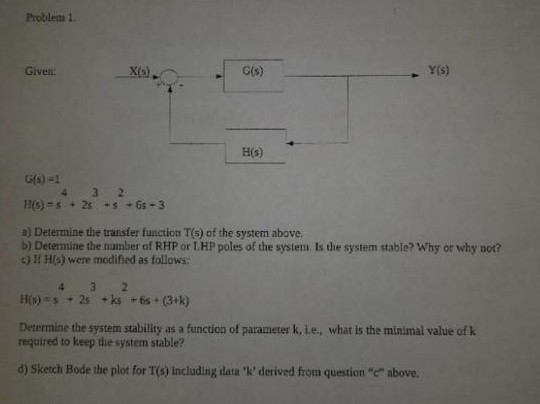Problem 1 Y(s) Given G(s) H(s) 0(s)-1 a) Determine the transfer function T(s) of the system above. b) Determine the mamber of RHP or L.HP poles of the system. Is tdhe system stable? Why or why no? c) H HG) were modified as follows. Determine the system stability as a function of parameter k, i.e, what is the minimal value of k required to keep the system stable? d) Sketch Bode the plot for T(s) including data 'k, derived from...

• ### 105- Problem #4 - Given the transfer function T(s)- ***(1+%o1+%0011+%00) A) Find the Poles and Zeros....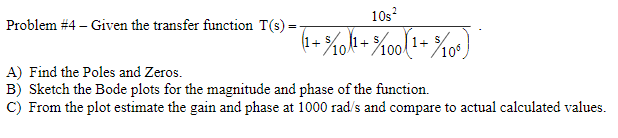105- Problem #4 - Given the transfer function T(s)- ***(1+%o1+%0011+%00) A) Find the Poles and Zeros. B) Sketch the Bode plots for the magnitude and phase of the function. C) From the plot estimate the gain and phase at 1000 rad/s and compare to actual calculated values.

• ### Given an input signal (t)nd the output signal is y(t)-(e2 e)u(t), compute the transfer function H(s)....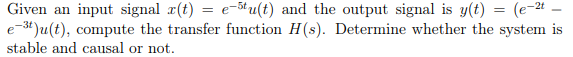Given an input signal (t)nd the output signal is y(t)-(e2 e)u(t), compute the transfer function H(s). Determine whether the system is stable and causal or not.

• ### i. For the transfer function s+2 T(S) - 2 +9 a. find the locations of the...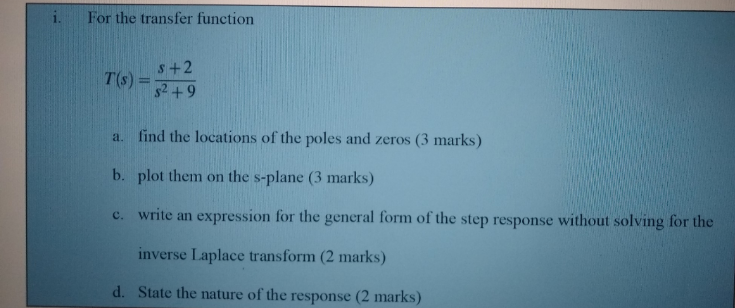i. For the transfer function s+2 T(S) - 2 +9 a. find the locations of the poles and zeros (3 marks) b. plot them on the s-plane (3 marks) c. write an expression for the general form of the step response without solving for the inverse Laplace transform (2 marks) d. State the nature of the response (2 marks)

• ### Problem 1: The impulse response ht) for a particular LTI system is shown below hit) Be5e (4 cos(3...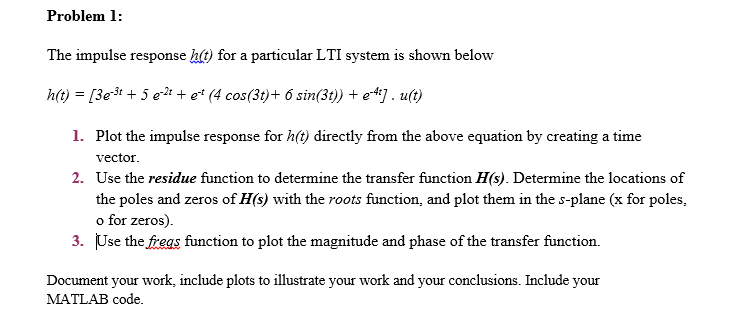Problem 1: The impulse response ht) for a particular LTI system is shown below hit) Be5e (4 cos(3t)+ 6 sin(3t) e. u(t) 1. Plot the impulse response for h(t) directly from the above equation by creating a time vector 2. Use the residue function to determine the transfer function H(s). Determine the locations of the poles and zeros of H(s) with the roots function, and plot them in the s-plane (x for poles, o for zeros). Use the freas function...

• ### Find poles and zeros, find system order, find the differential equation model. #7) Find X(s) and...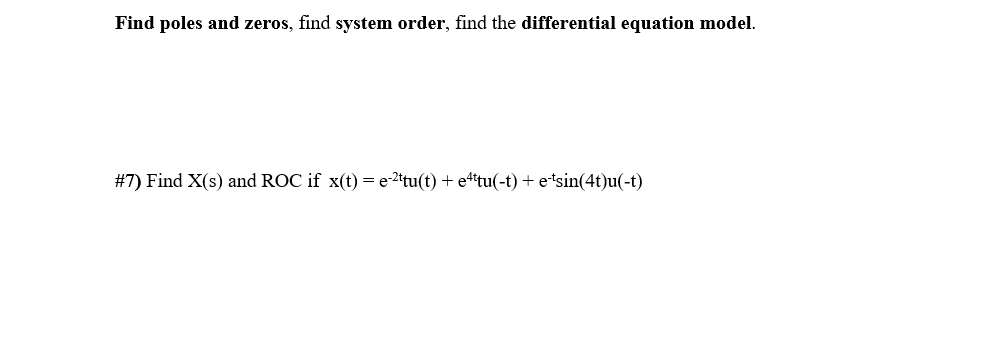Find poles and zeros, find system order, find the differential equation model. #7) Find X(s) and ROC if x(t) = e-Itu(t) + ettu(-t) + e'sin(4t)u(-t)

• ### 1. (20 points). A transfer function has the following zeros and poles: zero at s=-105 and s= poles at s-100 and s--1000. The magnitude of the transfer function at ω= 105 rad/s is equal 100. Find the...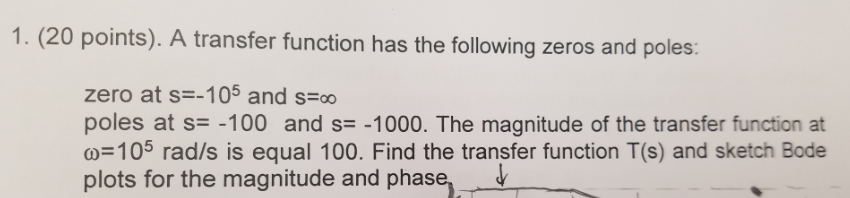1. (20 points). A transfer function has the following zeros and poles: zero at s=-105 and s= poles at s-100 and s--1000. The magnitude of the transfer function at ω= 105 rad/s is equal 100. Find the transfer function T(s) and sketch Bode plots for the magnitude and phase, ˇ 1. (20 points). A transfer function has the following zeros and poles: zero at s=-105 and s= poles at s-100 and s--1000. The magnitude of the transfer function at ω=...

• ### Theroot-locus design method (d) Gos)H(s)2) 5.5 Complex poles and zeros. For the systems with an open-loop transfer function given below, sketch the root locus plot. Find the asymptotes and their angle...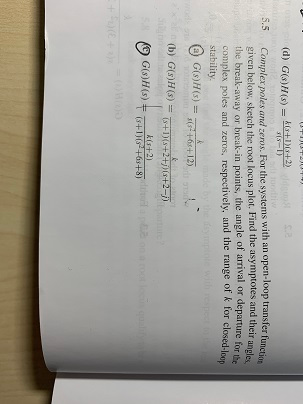Theroot-locus design method (d) Gos)H(s)2) 5.5 Complex poles and zeros. For the systems with an open-loop transfer function given below, sketch the root locus plot. Find the asymptotes and their angles. the break-away or break-in points, the angle of arrival or departure for the complex poles and zeros, respectively, and the range of k for closed-loop stability 5 10ん k(s+21 (d) Gos)H(s)2) 5.5 Complex poles and zeros. For the systems with an open-loop transfer function given below, sketch the root...